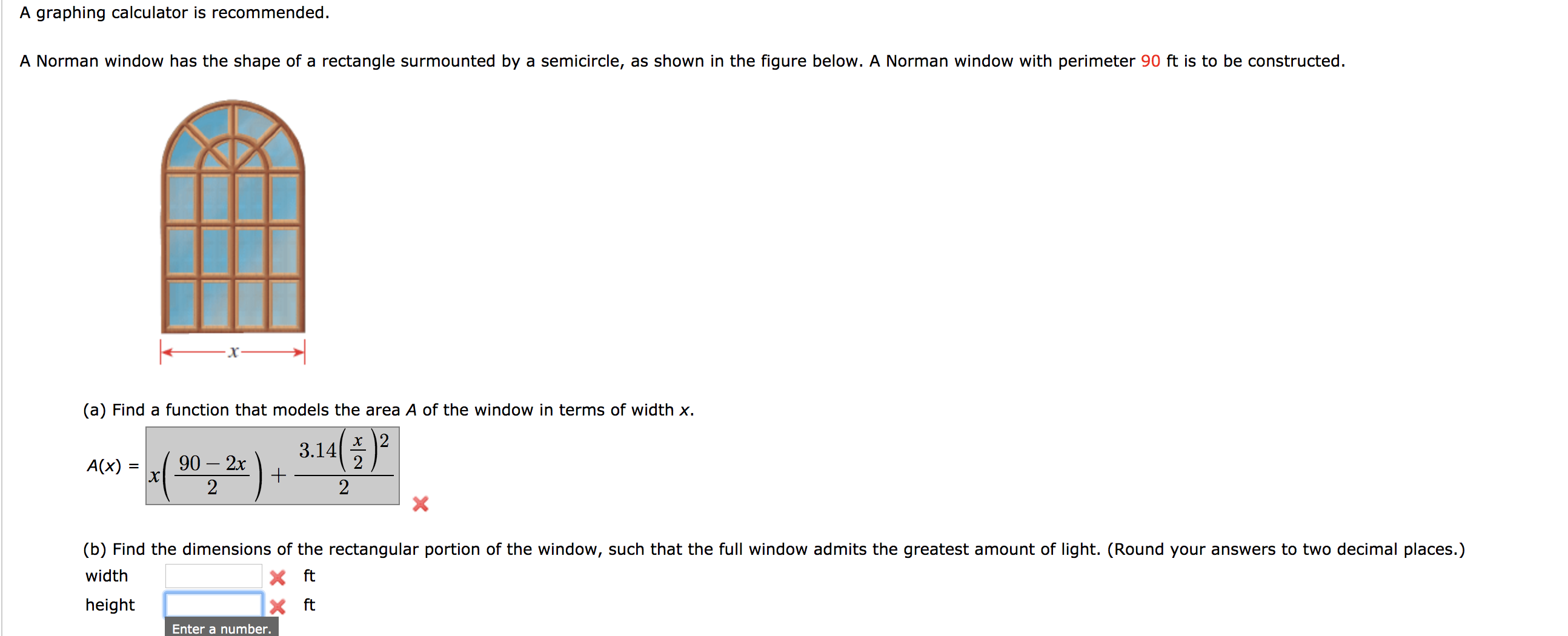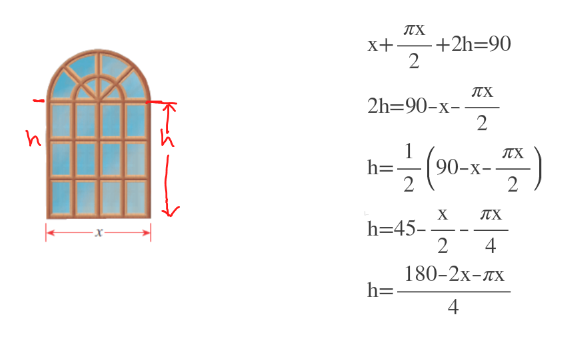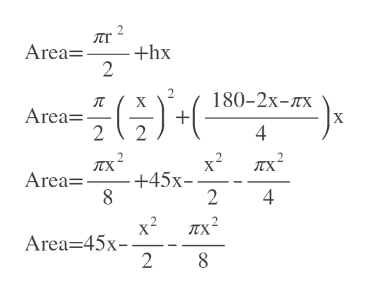# A graphing calculator is recommended.A Norman window has the shape of a rectangle surmounted by a semicircle, as shown in the figure below. A Norman window with perimeter 90 ft is to be constructed(a) Find a function that models the area A of the window in terms of width xх |23.1490 2xхA(x)+22X(b) Find the dimensions of the rectangular portion of the window, such that the full window admits the greatest amount of light. (Round your answers to two decimal places.)widthftX ftheightEnter a number.

Questionhelp_outlineImage TranscriptioncloseA graphing calculator is recommended. A Norman window has the shape of a rectangle surmounted by a semicircle, as shown in the figure below. A Norman window with perimeter 90 ft is to be constructed (a) Find a function that models the area A of the window in terms of width x х |2 3.14 90 2x х A(x) + 2 2 X (b) Find the dimensions of the rectangular portion of the window, such that the full window admits the greatest amount of light. (Round your answers to two decimal places.) width ft X ft height Enter a number. fullscreen
check_circleExpert Solution
Step 1

Diameter of the semi circle = x

Upper circumeference =pi*r= pi(x/2)=(pi*x)/2

Step 2

Let height of the rectangle part = h

We find h in terms of x.help_outlineImage Transcriptionclose-+2h=90 X+ 2 2h 90-x 2 (90-x-- TTX h= 2 2 X h=45 2 4 180-2x-Tx h= 4 Ks fullscreen
Step 3

We add the area of the semi circle with the area of the rectan...help_outlineImage TranscriptioncloseArea= +hx 2 2 180-2x-TX X Area= 4 x2 -+45x- 2 Area= 4 2 Area 45x- 2 fullscreen

### Want to see the full answer?

See Solution

#### Want to see this answer and more?

Solutions are written by subject experts who are available 24/7. Questions are typically answered within 1 hour*

See Solution
*Response times may vary by subject and question
Tagged in

### Other